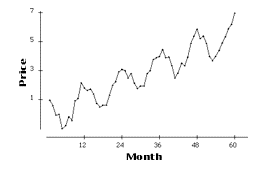### CFA Practice Question

There are 985 practice questions for this topic.

### CFA Practice Question

Consider the following time plot of the price of a commodity (in dollars) for each month of a 60-month period.Which of the following is (are) true about the time plot?

I. The increase in the price of the commodity from month 1 to month 60 is \$7.
II. The time plot displays a trend.